# Conditional formatting based on a formula part IIConditional formatting with a formula allows you to use Excel formulas to create complex formatting rules. In this article we will show you more examples of this amazing Excel feature.

To utilize Excel’s conditional formatting with a formula option you proceed as follows:

1. Select the range where the formatting will be applied.
2. After selecting Conditional Formatting from the Home Tab select New Rule.
3. Choose Use a Formula.
4.  With cell in upper left-hand corner of selected range type in a formula starting with =.
5. This formula will copy across and down selected range (watch your \$ signs!) Whenever the copied formula is True, the desired format will be used.

The file Beckermarch2020.xlsx contains three examples of conditional formatting with a formula. In each worksheet we have monthly sales of a product in three different stores. We want to use conditional formatting with a formula to create three different formats.

1. Highlight in yellow for each store their best sales month.
2. Highlight in yellow for each month the store with the largest sales.
3. Highlight in yellow the largest number of units sold over all months and stores.

Highlight in yellow for each store their best sales month.

As shown in Figure 1 and the worksheet Best for Store, the largest sales for each store is highlighted.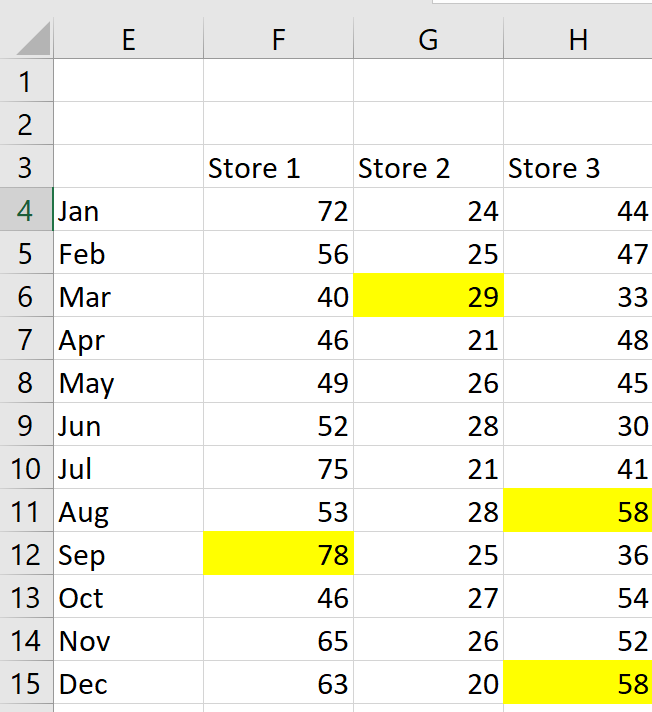Figure 1: Largest sales for each store is highlighted

To create this format we selected the cell range F4:H16 and then selected Conditional Formatting from the Home tab. Then, we selected New Rule Use a Formula and filled in the dialog box as shown in Figure 2.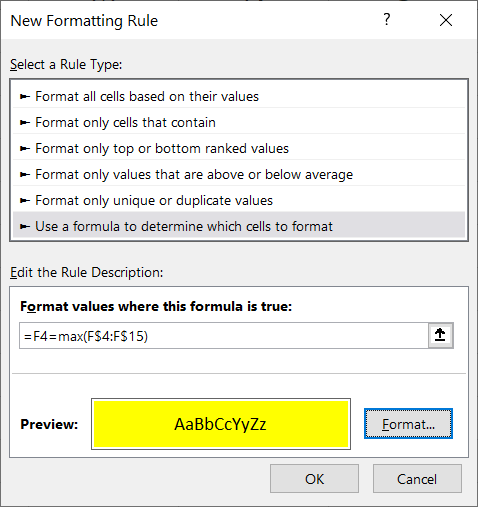Figure 2: Formula that highlights largest sales for each store.

As Excel copies this formula to each cell in the selected range, the number in each cell is compared to the largest number in the cell’s column. You need to dollar sign the 4 and 15, so that when the formula is copied down you always compare the current cell to the largest number in the same column in rows 4-15. Then the cell is highlighted if it equals the largest number in its column.

Highlight in yellow for each month the store with the largest sales.

As shown in Figure 3 and the worksheet Best for Month, the largest sales for each month is highlighted.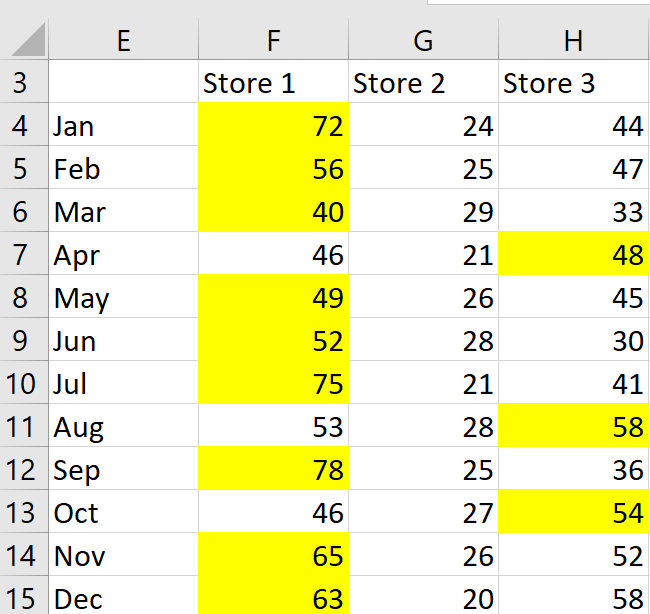Figure 3: Largest sales for each month is highlighted

To create this format we selected the cell range F4:H16 and then selected Conditional Formatting from the Home tab. Then, we selected New Rule Use a Formula and filled in the dialog box as shown in Figure 4.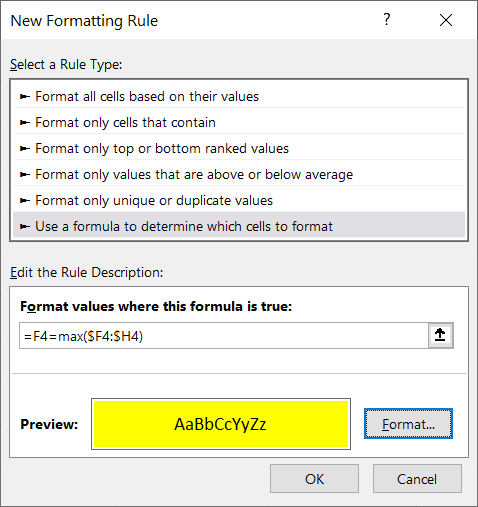Figure 4: Formula that highlights largest sale for each month

When copied to the range F4:H16 this formula compares each cell to the largest number in the cell’s row and highlights the cell in yellow if and only if the cell is the largest number in its row.

Highlight in yellow the largest number of units sold over all months and stores.

As shown in Figure 5 and the worksheet Best Overall, the largest sales overall is highlighted.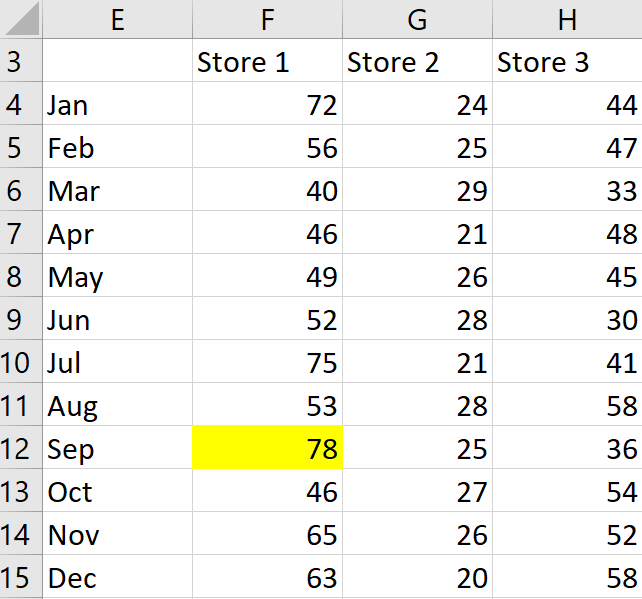Figure 5: Largest sales overall is highlighted

To create this format we selected the cell range F4:H16 and then selected Conditional Formatting from the Home tab. Then we selected New Rule Use a Formula and filled in the dialog box as shown in Figure 6.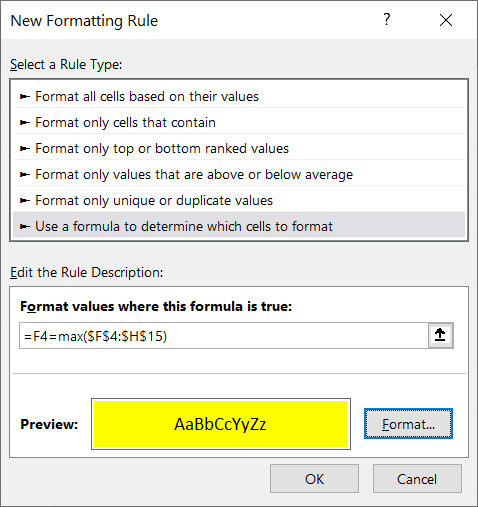Figure 6: Formula that highlights largest sales overall

When copied to the range F4:H16 this formula compares each cell to the largest number in range F4:H16 and highlights the cell in yellow if and only if the cell is the largest number in the range F4:H16.

For more complex examples of Conditional Formatting, read part 3 of the series.